AP State Syllabus AP Board 9th Class Maths Solutions Chapter 13 Geometrical Constructions Ex 13.1 Textbook Questions and Answers.

## AP State Syllabus 9th Class Maths Solutions 13th Lesson Geometrical Constructions Exercise 13.1Question 1.
Construct the following angles at the initial point of a given ray and justify the construction.
a) 90°
Solution: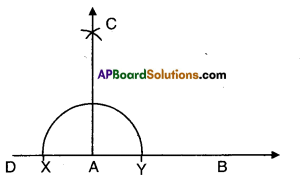• Let AB be the given ray.
• Produce BA to D.
• Taking A as centre draw a semi circle with some radius.
• With X and Y as Center draw two intersecting arcs of same radius.

Or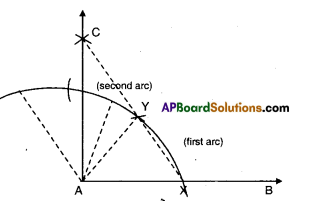|

• Let $$\overrightarrow{\mathrm{AB}}$$ be the given ray.
• With A as centre draw an arc of any radius.
• Mark off two equal arcs from X as shown in the figure with the same radius taken as before.
• Bisect the second segment.
• Join the point of intersection of above arcs, with A.
• ∠BAC is the required right angle.
• Join the point of intersection ‘C’ and ‘A’.
• ∠BAC = 90°

In ΔAXY; ∠YAX = 60° and
in ΔAYC ∠YAC = 30° ∠BAC = 90°b) 45°
Solution:
Steps:

• Construct 90° with the given ray AB.
• Bisect it from ∠BAD = 45°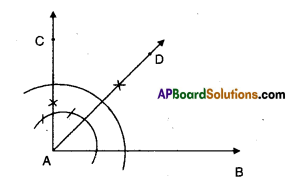Or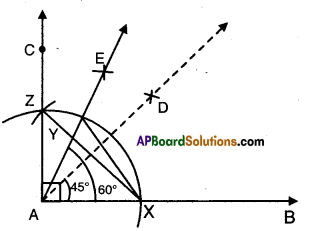Steps:

• Construct ∠BAC = 60°
• Bisect ∠BAC = ∠DAC = 30°
• Bisect ∠DAC such that ∠DAE = ∠FAC = 15°
• ∠BAE=45°

ΔAXZ is equilateral
and ∠YAZ = 15°
∴∠XAY = 45°Question 2.
Construct the following angles using ruler and compass and verify by measuring them by a protractor.
a) 30°
Solution: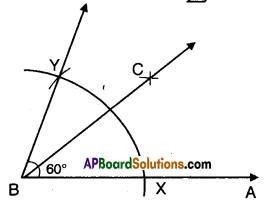• Construct ∠ABY = 60°
• Bisect ∠ABY = 60°
• Such that ∠ABC = ∠CBY = 30°

b) 22$$\frac{1}{2}^{\circ}$$
Solution: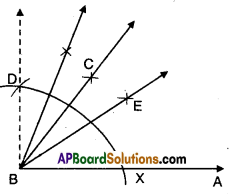• Construct ∠ABD = 90°.
• Bisect ∠ABD such that ∠ABC = ∠CBD = 45°
• Bisect ∠ABC such that
∠ABE = ∠EBC = 22$$\frac{1}{2}^{\circ}$$c) 15°
Solution: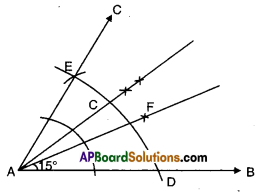Steps of construction : ,

• Construct ∠BAE = 60°
• Bisect ∠BAE such that ∠BAC = ∠CAE = 30°
• Bisect ∠BAC such that ∠BAF = ∠FAC = 15°

d) 75°
Solution: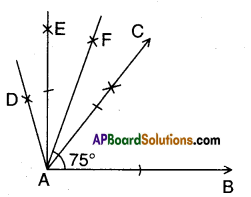Steps of construction :

• Construct ∠BAC = 60°
• Bisect ∠CAD such that ∠BAE = 90°
• Bisect ∠CAE such that ∠BAF = 75°e) 105°
Solution: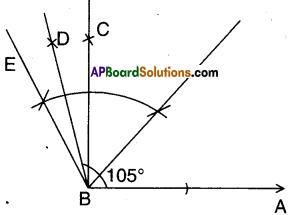Steps of construction:

• Construct ∠ABC = 90°
• Construct ∠CBE = 30°
• Bisect ∠CBE such that the angle formed ∠ABD = 105°

f) 135°
Solution: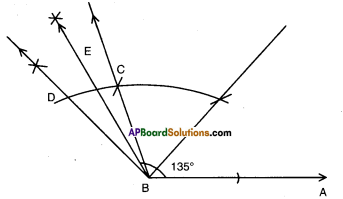Steps of construction:

• Construct ∠ABC = 120°
• Construct ∠CBD = 30°
• Bisect ∠CBD such that the angle formed ∠ABE = 135°Question 3.
Construct an equilateral triangle, given its side of length of 4.5 cm and justify the constraction.
Solution:
A.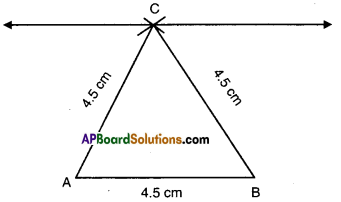• Draw a line segment AB = 4.5 cm.
• With B and A as centres draw two arcs of radius 4.5 cm meeting at C.
• Join C to A and B.
• ΔABC is the required triangle.

Justification:
In ΔABC
AB = ∠C ⇒ ∠C = ∠B
Also AB = BC ⇒ ∠C = ∠A
Hence ∠A = ∠B = ∠C
But ∠A + ∠B + ∠C = 180°
∴ ∠A = ∠B = ∠C = $$\frac{180^{\circ}}{3}$$ = 60°Question 4.
Construct an isosceles triangle, given its base and base angle and justify the construction. [Hint: You can take any measure of side and angle]
Solution:
A.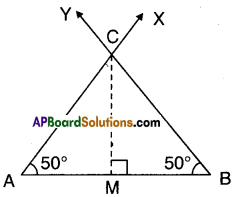Steps:

• Draw a line segment AB of any given length.
• Construct ∠BAX and ∠ABY at A and B such that ∠A = ∠B.
• $$\overrightarrow{\mathrm{AX}}$$ and $$\overrightarrow{\mathrm{BY}}$$ will intersect at C.
• ΔABC is the required triangle.

Justification:
Drop a perpendicular CM to AB from C.
Now in ΔAMC and ΔBMC
∠AMC = ∠BMC [Right angle]
∠A = ∠B [Construction]
CM = CM (Common)
∴ ΔAMC ≅ ΔBMC
⇒ AC = BC [CPCT]